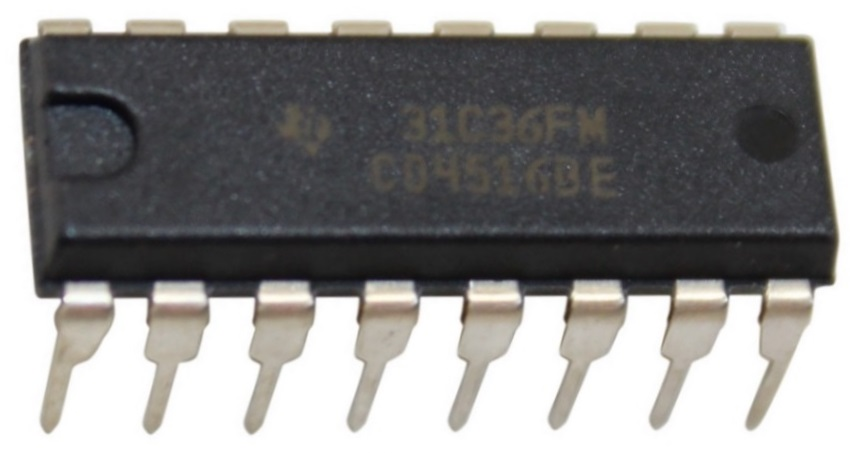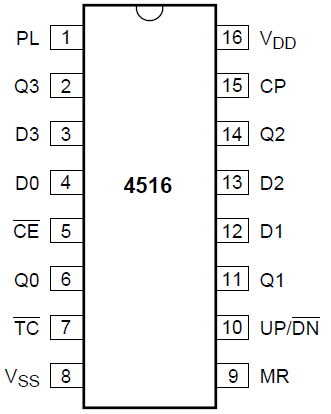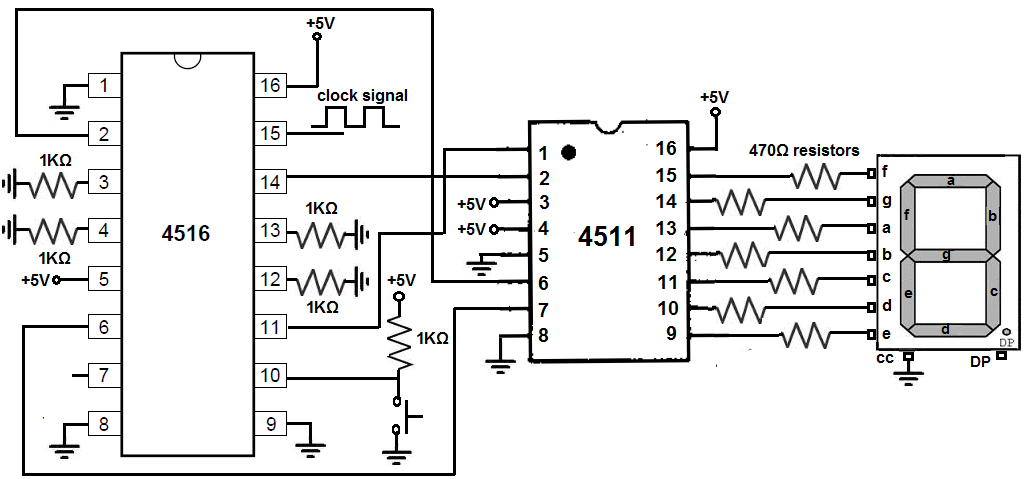﻿ How to Build a 4516 Binary Up/Down Counter Circuit ﻿# How to Build a 4516 Binary Up/Down Counter CircuitIn this circuit, we will build a binary up/down counter with a 4516 chip.

A binary up/down counter chip is a chip which can count up or down in binary values incrementing or decrementing by 1 at a time.

So when the binary counter is in count up mode, it counts up from 0 to 1 to 2 to 3...all the way to 15, in binary values. After it reaches 15, it then goes to 0, starting all over again.

When the binary counter is count down mode, it counts in reverse. So if it's at 15, it then goes to 14, then 13, then 12, all the way down to 0. It then goes back to 15 and counts back to 0. And the cycle goes on and on.

A binary counter counts in binary. The 4516 chip has 4 data inputs. So it counts from 0000 to 1111 in count up mode. And from 1111 to 0000 in count down mode.

In this circuit, we will use a binary counter and connect it to a 4511 BCD to 7 segment display decoder. Since the binary counter increments by 1 each clock cycle in count up mode, we will see the decimal digit on the 7 segment display count up each cycle. So it'll initially display 0, then 1, 2, 3, 4, 5, 6, 7, 8, and then 9. It cannot display a digit above the value of 9. We can then switch to count down mode, and it will display 9, then 8, then 7, 6, 5, 4, 3, 2, 1, 0.

So this circuit uses a binary counter to display the decimal digits in sequential order, each up or down. It shows the practical use of binary counters and it enables you to count up or down without having to use a microcontroller. So we can just use basic chips to count sequentially and physically show the results through a 7 segment LED display. It saves us to have to connect to computers and have to write a program. So it's very useful.

Components

• 4516 Binary Up/Down Counter IC
• 4511 BCD to 7 Segment Display Decoder IC
• 7 segment display
• 7 470Ω resistors
• 4 1KΩ resistors
• Pushbutton
• Clock Signal Generator

The 4516 binary up/down counter can be gotten very cheaply for about \$0.50 on ebay.

The 4516 is a 16-pin chip. The pinout of the 4516 is shown below.To power the chip, VDD, pin 16 to 5V and VSS, pin 8, connects to ground. The 4516 chip is capable of handling up to 18V.

Pin 1 is the preload pin. In normal operation, this pin is kept LOW or grounded. When HIGH, the chip functions in parallel load input.

D3, D2, D1, and D0, pins 3, 13, 12, and 4, are the data input pins.

B8, B4, B2, and B1 are the output pins. These are the pins we connect to the data input pins of the 4511 chip.

Pin 5 is the CE pin. This stands for count enable. Placing this pin LOW allows the chip to count. This pin must be held LOW to allow counting. Placing this pin HIGH stops the count.

Pin 7 is the TC pin, which is the terminal count pin. We will leave this pin unconnected because it is not necessary for the counting operation of the chip. It basically can serve as a status indicator to see the values of the output pins and whether the chip is in up or down mode. We leave this pin unconnected or floating.

Pin 9 is the MR or Reset pin. It resets the count back to 0000. This pin is active HIGH. So in normal operation, it is connected LOW for the count operation to proceed. When connected HIGH, the output pins, Q0 to Q3, get reset to 0000.

Pin 10 is the Up/DN pin. This pin controls whether the chip counts up (from 0000 to 1111) or counts down in reverse (from 1111 to 0000). When the pin is HIGH, it is count up mode. When the pin is LOW, it is count down mode.

Pin 15 is the clock pin. The 4516 chip is a chip that works on the clock signal. For each clock signal input, the chip counts up or down by 1. Without a clock signal, the chip cannot work, so we must feed a clock signal into pin 15.

### 4516 Binary Up/Down Counter Circuit

The binary up/down counter circuit we will build with a 4516 chip is shown below.First, we power each of the chips. We do this by providing VDD with +5V to the 4511 and 4516 chips. The VDD pin is pin 16 for both chips. Then we connect VSS to ground on both chips. VSS is pin 8 for both chips.

For the 4511 is a BCD to 7 segment display decoder chip. It takes a binary value and displays the equivalent decimal digit on a 7 segment display. We're not going into great depth about connecting it. If you would like to see an in-depth circuit on how to connect the 4511, see 4511 BCD to 7 Segment Display Decoder Circuit.

But for a quick overview to connecting it here, we connect pin 3, the LT, the lamp test pin, HIGH. If LOW, all outputs will be turned. It's used for testing purposes to see if all outputs turn on. Pin 4 is the blanking. We connect this pin HIGH so that all the outputs don't go LOW. This pin is clear all outputs to a LOW state. Pin 5 is the Strobe pin. We connect this pin LOW to strobe the outputs to the 7 segment LED Display. If HIGH, it simply stores it but doesn't transfer it to a device. Pins D, C, B, and A, pins 6, 2, 1, 6, are the input pins. The output of the 4516 chip will connect into these input pins. The output pins of the 4511 is a, b, c, d, e, f, and g are the output pins. To each of these pins, we connect an LED along with a current-limiting 470Ω resistor.

Now for the 4516 binary up/down counter chip, we connect the preload pin to ground for normal counting operation.

We connect the CE or count enable pin, HIGH. This enables counting. If connected LOW, counting, either up or down, is inhibited.

Pin 7 is the TC pin. We leave this pin unconnected, since it doesn't affect the counting operation. It's just serves as a status indicator.

We connect the MR pin, pin 9, to ground to disable the clearing feature of all outputs. If connected HIGH, all outputs would go to a LOW state.

We connect the Up/DN pin, pin 10 to a pull-up resistor. With a pull-resistor, when the pushbutton is unpressed, the pin will be in a HIGH state. So unpressed, the pin is in a HIGH state. This puts the chip in count up mode. So it goes from 0 to 1 to 2...15...then back to 0. When this pushbutton is pushed, the pin now goes to a LOW state, putting the chip in count down mode. The chip will now count from 15 to 14 to 13...then back to 15. So this pushbutton or any switch you would like to use gives us this flexibility to switch from up to down counting.

The CP pin, pin 15, the clock pin. This pin needs a constant clock pulse in order for the circuit to work. The 4516 chip works on a clock signal. For each clock signal, it either increases or decreases the count by 1, depending on whether the chip is in up or down count mode. This clock signal can be given in a variety of ways. It can given with a 4046 VCO chip output. It can given from the output of a 555 timer in bistable mode. If you have function generator, you can also connect it to the pin and put a fairly low frequency signal into the clock. The generator should be put in square wave mode. You can calculate the time each cycle will take by taking the frequency and plugging it into the formula, T= 1/f, where T is the period in unit seconds and f is the frequency of the signal. You want a faily low signal, such as 1 Hz. 1Hz produces a time period of 1 second. So each clock cycle would 1 second. So you can actually see the output. Otherwise, the display would change so fast, you would barely be able to catch anything. So there's many ways of doing this.

D3, D2, D1, and D0 are the data input pins for the 4516 chip. They connect to ground through a resistor. We use a 1KΩ resistor for each of the data pins. When the circuit first starts out, it starts at 0000 for the value of the data pins and then counts up or down depending on the mode. So these data inputs need no data for us to enter it. It just automatically does so. There are some binary counters that allow presettable data inputs, but the 4516 does not.

The output pins, Q3, Q2, Q1, and Q0, connect to the data input pins of the 4511 chip. So that as the value is incremented or decremented by 1 each clock cycle, the new data is input into the 4511 chip, which then displays it on the 7 segment LED display. So the 4516 gives the data counting up or down by 1 each cycle and then feeds that data into the 4511 chip, which then displays it on the 7 segment LED display.

So this is how a binary up/down counter with a 4516 chip works.

Related Resources